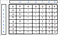# Printing Longest Common Subsequence — Day 58(Python)Photo by Tolga Ulkan on Unsplash

Today’s question is an additional step to the LCS question. In case you require a refresher on solving LCS, you can follow this link.

Printing Longest Common Subsequence

Given two sequences, print the longest subsequence present in both of them.

Examples:
LCS for input Sequences “ABCDGH” and “AEDFHR” is “ADH” of length 3.
LCS for input Sequences “AGGTAB” and “GXTXAYB” is “GTAB” of length 4.

Let us take an example and solve this problem.

Before running through an example, let us recall the code for solving the LCS problem using the Bottom-Up approach.

`class FindLongest:    def longestCommonSubsequence(self, text1: str, text2: str) -> int:        memo = [[float('-inf') for j in range(len(text2)+1)]for i in range(len(text1)+1)]        for i in range(len(memo)):            for j in range(len(memo)):                if i == 0 or j == 0:                    memo[i][j] = 0                elif text1[i-1] == text2[j-1]:                    memo[i][j] = 1 + memo[i-1][j-1]                else:                    memo[i][j] = max(memo[i-1][j], memo[i][j-1])        return memo[-1][-1]`

Okay, let’s move with the example. Let the input strings be “AGGTAB” and “GXTXAYB”. When we run the above code for the given input string, let us see the content in the matrix.Content of DP matrix for LCS

As the answer to the LCS problem, we return the value present in the last cell. In this case, our answer is 4 for LCS.

We will initialize an answer variable where we will be storing the result of LCS. We will initialize another two variables, “i” and “j” that can keep track of row and column in the matrix. The rows represent characters in string1 and columns represent the characters in string2.

To print the LCS, we will start from the last cell. We will check if characters at ith index in string1 and the jth index in string2 are equal. If they are equal, we will append it to our answer variable and then move diagonally upward. If not, we will check which cell has the higher value. Is it the one to the left or the one to the top? Accordingly, we will either decrement the value of i or j. Why are we comparing or taking the higher value? When we were trying to fill the matrix, if the characters were unequal, we take the maximum cell value among its left or top cell value.

`memo[i][j] = max(memo[i-1][j], memo[i][j-1])`

Let us see what we get when we traverse using the above logic.

In the end, we will reverse the value in the answer variable.

Let us look into the code snippet.

`class FindLongest:    def longestCommonSubsequence(self, text1: str, text2: str) -> int:        memo = [[float('-inf') for j in range(len(text2)+1)]for i in range(len(text1)+1)]        for i in range(len(memo)):            for j in range(len(memo)):                if i == 0 or j == 0:                    memo[i][j] = 0                elif text1[i-1] == text2[j-1]:                    memo[i][j] = 1 + memo[i-1][j-1]                else:                    memo[i][j] = max(memo[i-1][j], memo[i][j-1])        i = len(memo)-1        j = len(memo)-1        ans = ""        while(i >= 0 and j >= 0):            if text1[i-1] == text2[j-1]:                ans += text1[i-1]                i -= 1                j -= 1            else:                if memo[i-1][j] > memo[i][j-1]:                    i -= 1                else:                    j -= 1        return(ans[::-1])`

Complexity analysis.

Time Complexity

We are traversing through a 2D array of size M*N, hence the time complexity is O(M*N), where M is the size of string1 and N is the size of string2.

Space Complexity.

We are creating a 2D array of size M*N, hence the space complexity is O(M*N), where M is the size of string1 and N is the size of string2.

Link to Aditya Verma’s video

## More from Annamariya Tharayil

Software Engineer. Find me @ www.linkedin.com/in/annamariya-jt